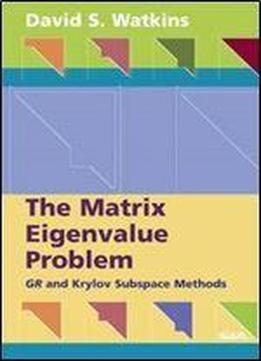# The Matrix Eigenvalue Problem: Gr And Krylov Subspace Methods by David S. Watkins / 2018 / English / PDF

This book presents the first in-depth, complete, and unified theoretical discussion of the two most important classes of algorithms for solving matrix eigenvalue problems: QR-like algorithms for dense problems and Krylov subspace methods for sparse problems. The author discusses the theory of the generic GR algorithm, including special cases (for example, QR, SR, HR), and the development of Krylov subspace methods. Also addressed are a generic Krylov process and the Arnoldi and various Lanczos algorithms, which are obtained as special cases. The chapter on product eigenvalue problems provides further unification, showing that the generalized eigenvalue problem, the singular value decomposition problem, and other product eigenvalue problems can all be viewed as standard eigenvalue problems. The author provides theoretical and computational exercises in which the student is guided, step by step, to the results. Some of the exercises refer to a collection of MATLAB programs compiled by the author that are available on a Web site that supplements the book. Audience: Readers of this book are expected to be familiar with the basic ideas of linear algebra and to have had some experience with matrix computations. This book is intended for graduate students in numerical linear algebra. It will also be useful as a reference for researchers in the area and for users of eigenvalue codes who seek a better understanding of the methods they are using. Contents: Preface Chapter 1: Preliminary Material Chapter 2: Basic Theory of Eigensystems Chapter 3: Elimination Chapter 4: Iteration Chapter 5: Convergence Chapter 6: The Generalized Eigenvalue Problem Chapter 7: Inside the Bulge Chapter 8: Product Eigenvalue Problems Chapter 9: Krylov Subspace Methods Bibliography Index.

views: 183